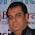## Bitwise operators in c with example

Bitwise used for manipulation of data in bit level.

 Operator Function ` bitwise NOT << left shift >> right shift & bitwise AND ^ bitwise XOR | bitwise OR &= bitwise AND assign ^= bitwise XOR assign |= bitwise OR assign

Bitwise not applicable for float, double and char.

Syntax:-

syntax for & | and ^

value1 bitwise value2

Example:-
2 & 3
num1 & num2

Here value1 and value2 must be integer data type

syntax for >> and <<

value bitwise number_of_shift

Example:-
6 >> 2
num >> 3

Here value and number_of_shift  must be integer data type

Note:-
# Left shift by 2 is equal to multiply by 2
# Right shift by 2 is equal to division by 2

Example:-

Program for shift left

Output:-
Enter a number
5

5 is in binary 101 when we shift left we put zero at right side. Here we shifting by 2 so we put 2 zero at right side 10100 ie 20

Previous Code:-
Logical operators

List Code:-
C Codes

Next Code:-
if decision making statement

C Programs
List of c Programs

### One Comment

1.Thanks for sharing valuable information.This post gives required information to the visitor
sap training institute in Noida
sap training institute in Delhi